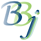# Date Input

## Description

In BBj 4.0 and higher, the date input control (BBjInputD) and a new version of the JUL() function can be used to convert a human readable date to a Julian day number.

## Date Formats

There are three basic date formats in use around the world, based on the order of the Month, Day, and Year. Within these basic formats, there are local differences in the preferred separator, (usually "-", "/" or "."), whether years are shown as four digits or two digits, and whether 1-digit month and day numbers are padded to two digits with a leading "0"). In Europe, most countries use day/month/year format. The United States uses month/day/year. China, Japan, and Korea use year/month/day (the ISO standard, normally formatted as YYYY-MM-DD).

The date parser used by the BBjInputD control and the JUL() function interprets date strings based on the date mask. For example, if the date mask is %Mz/%Dz/%Yz (US format, the BBj default setting), the date is parsed with the understanding that it is in month/day/year order. Dates are accepted in all reasonable numeric formats, with or without delimiters. For example, assuming that today is September 15, 2012, this is how various inputs would be interpreted:

 Entry YMD (ISO) MDY (US) DMY (EU) 1 A single digit is always interpreted as a day number within the current month, so this would be September 1, 2012. 12 Two digits are always interpreted as a day number within the current month, so this would be September 12, 2012. 112 Three digits are interpreted as a 1-digit month number followed by a 2-digit day number, so this would be January 12, 2012. Three digits are interpreted as a 1-digit day number followed by a two-digit month number, so this would be 1 December 2012. 1004 Four digits are interpreted as MMDD, so this would be October 4, 2012. Four digits are interpreted as DDMM, so this would be 10 April 2012. 020304 Six digits are interpreted as YYMMDD, so this would be March 4, 2002. Six digits are interpreted as MMDDYY, so this would be February 3, 2004. Six digits are interpreted as DDMMYY, so this would be 2 March 2004. 8 digits Eight digits are interpreted as YYYYMMDD. For example, 20040612 is June 12, 2004. Eight digits are interpreted as MMDDYYYY. For example, 06122004 is June 12, 2004. Eight digits are interpreted as DDMMYYYY. For example, 06122004 is 6 December 2004. 12/6 Two numbers separated by any valid delimiter is interpreted as MM/DD, so this would be December 6, 2012. (Note: All characters except for letters and digits are considered valid delimiters.) Two numbers separated by any delimiter is interpreted as DD/MM, so this would be 12 June 2012. 3/4/5 April 5, 2012 March 4, 2005 3 April 2005

## Month and Week Formats

In BBj 16.0 and higher, BBj date input and date parsing modules accept year/month, month/year, year/week, and week/year formats.  When the mask contains only %Y and %M, and no %D, input of various lengths is interpreted as follows:

 Entry YM MY 1 A single digit is interpreted as the specified month in the current year, so this would be January 2012. 12 Two digits are interpreted as the specified month in the current year, so this would be December 2012. 112 Three digits are interpreted as a two digit year followed by a one digit month, so this would be February 2011. Three digits are interpreted as a one digit month followed by a two digit year, so this would be January 2012. 1004 Four digits are interpreted as YYMM, so this would be April 2010. Four digits are interpreted as MMYY, so this would be October 2004. 5 digits A five digit date entry is always considered invalid. 020304 Six digits are interpreted as YYYYMM, so this would be April, 0203. Six digits are interpreted as MMYYYY, so this would be February, 0304. 7 or more digits Seven or more digits are considered invalid. 12/6 Two numbers separated by any valid delimiter are interpreted as YY/MM, so this would be June 2012. Two numbers separated by any valid delimiter are interpreted as MM/YY, so this would be December 2006..

## Week Numbers

It is useful to be able to enter a date based on a combination of week number in the year and day number in the week. A week-based date can be entered in the format {yyyy}Www{d} or {yyyy-}Www{-d}, where the parts in {} are optional. This is a subset of ISO standard 8601.

Some examples are:

 W10 The first day of the tenth week of the current year. W10-3 The third day of the tenth week of the current year. 2013W055 The fifth day of the fifth week of 2013. 3-W5-5 Equivalent to the previous example.

This ISO 8601 format, which explicitly includes a 'W', may be used with any date mask. In BBj 16.0 and higher, when the mask contains only %y, %w and optionally %d (all  lower case), input of various lengths is interpreted as follows:

 Entry yw, ywd wy 1 A single digit is interpreted as the specified week in the current year, so this would be week 1 in 2012. 12 Two digits are interpreted as the specified week in the current year, so this would be week 12 in 2012. 112 Three digits are interpreted as a two digit year followed by a one digit week, so this would be week 2 in 2011. Three digits are interpreted as a one digit week followed by a two digit year, so this would be week 1 in 2012. 1004 Four digits are interpreted as yyww, so this would be week 4 in 2010. Four digits are interpreted as wwyy, so this would be week 10 in 2004. 10043 For the ywd format, five digits are interpreted as YYWWD, so this would be day 3 of week 4 in 2010. 020304 Six digits are interpreted as yyyyww, so this would be week 4 in 0203. Six digits are interpreted as wwyyyy, so this would be week 2 in 0304. 12/6 Two numbers separated by any valid delimiter are interpreted as YY/MM, so this would be June 2012. Two numbers separated by any valid delimiter are interpreted as MM/YY, so this would be December 2006. 12/6/5 Three  numbers separated by any valid delimiter are interpreted as y/w/d, so this would be day 5 of week 6 in 2012. Invalid

Since the rules for calculating week numbers vary from country to country, the date parser refers to STBL("!LOCALE") to determine what rules should be used. Below are examples for January 12, 2005, using the sample program from BBjInputD::setLocale.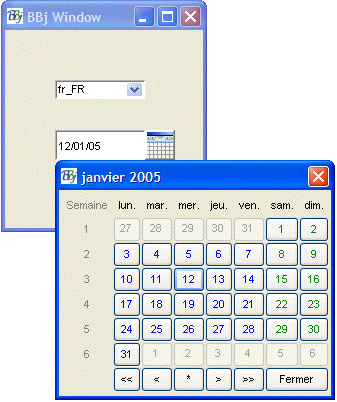In France, as in most of the EU, Monday is the first day of the week. France defines the first week of the year as being the week that includes January 1. Based on these rules, January 12, 2005 is 2005-W3-3 – the third day of the third week.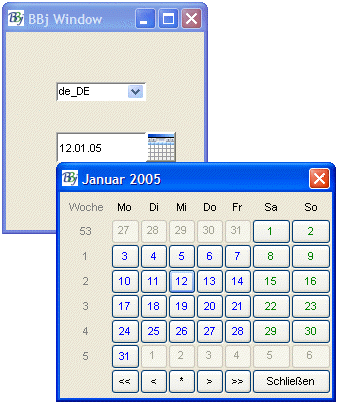In Germany, as in most of the EU, Monday is the first day of the week. Germany defines the first week of the year as being the week that includes January 4. Based on these rules, January 12, 2005 is 2005-W2-3 – the third day of the second week.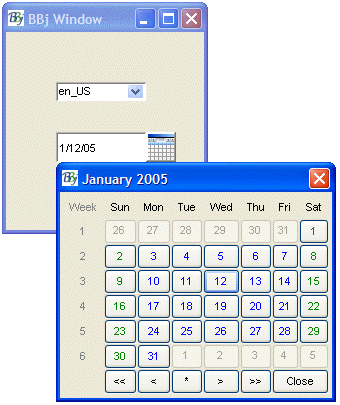The United States defines the first week of the year as being the week that includes January 1. Weeks start on Sunday in the United States. Based on these rules, January 12, 2005 is 2005-W3-4 – the fourth day of the third week.

## Popup Calendar

The user can pop up a calendar to pick a date interactively by pressing the POPUP hotkey from a BBjInputD control (see !EDIT in STBL Formats - BBj). The default setting for this hotkey is CTRL+P. Alternatively, the BBjInputD::calendar() method can be invoked to pop up a calendar, typically in response to user action like clicking on a toolbutton. The calendar is localized based on the current value of STBL("!LOCALE") (this can be overridden for a particular INPUTD control using the BBjInputD::setLocale() method).

For example, this image shows a popup calendar for Spanish (Mexico):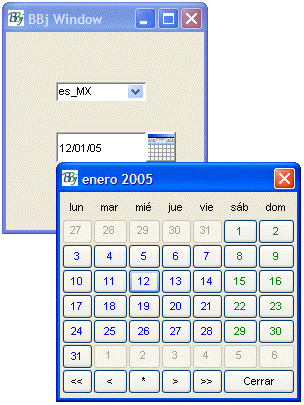Clicking on any date in the currently displayed month causes that date to be returned to the input area. Within the popup calendar, the arrow keys navigate between the buttons, the Page Up key goes to the next month, and the Page Down key goes to the previous month. The ENTER or SPACE key activates the current button, and the ESCAPE key exits without making any selection.

## Relative Dates

A date string consisting of + or - followed by an integer is interpreted as the specified number of days, weeks, or months before or after the current date, depending on the current mask.

## Two-Digit Years

Dates are typically entered using two-digit years, with the century portion being implied either by the context or by local understanding. For example, it would make sense to assume only dates prior to today in a "Date of Birth" field, so 10/10/10 would be interpreted as October 10, 1910. In other applications, it might make sense to assume only dates in the future, for example if entering new information for a long-term lease. Or you might want to assume that all years are in the current century, so that 01/01/01 is January 1, 2001 and 01/01/99 is January 1, 2099. Or you can define a sliding rule, where two-digit dates fall into a range from 80 years ago through 19 years in the future, or from 1970 through 2069. The date parser allows for all of these possibilities with the STBL("!YYDATE") global string. The default rule is -50/+49, which means that two-digit years entered in 2012 would be assumed to fall within the range from 1953 through 2052.

The STBL("!YYDATE") is not taken into account by the JUL() function that takes only numerical values, but is taken into account by the JUL() function that takes character string values, e.g. for a current year of 2011:

x\$=stbl("!YYDATE","+1")

print date(jul("010211"),"%Yd-%Mz-%Dz")

2011-01-02

print date(jul("010212"),"%Yd-%Mz-%Dz")

2012-01-02

print date(jul("010213"),"%Yd-%Mz-%Dz")

1913-01-02

print date(jul("070462"),"%Yd-%Mz-%Dz")

1962-07-04

## Custom Input Rules

If BBjInputD is unable to parse the user's input as a valid date, the text is left as typed by the user. An event handler can be written for the ON_FOCUS_LOST event to examine the control to see if it contains a valid date. If not, it can attempt to apply local rules for interpreting the user's input. See BBjInputD::isValid for a sample program that defines the locally defined special code "me" for "month end."